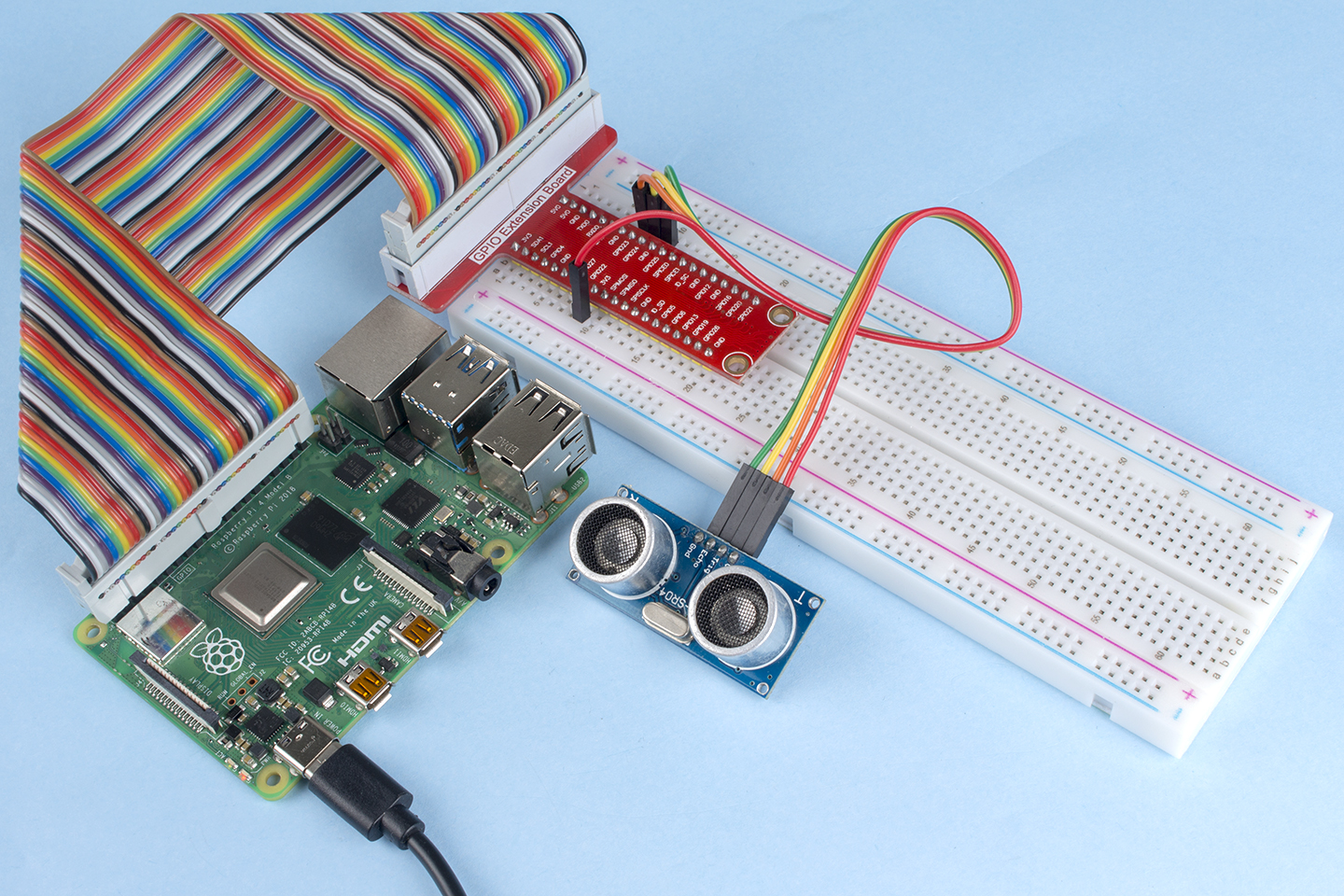# 2.2.5 Ultrasonic Sensor Module¶

## Introduction¶

The ultrasonic sensor uses ultrasonic to accurately detect objects and measure distances. It sends out ultrasonic waves and converts them into electronic signals.

## Components¶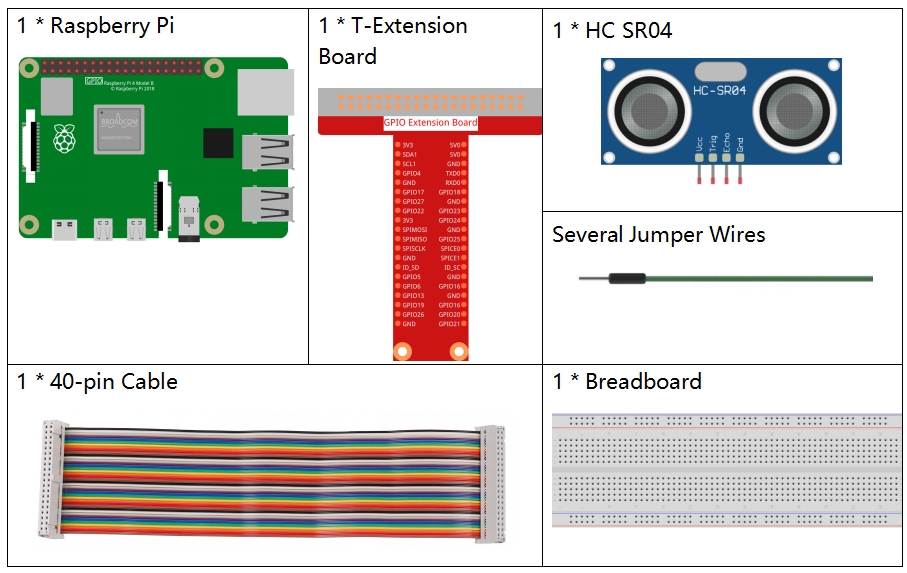## Principle¶

Ultrasonic

Ultrasonic ranging module provides 2cm - 400cm non-contact measurement function, and the ranging accuracy can reach to 3mm. It can ensure that the signal is stable within 5m, and the signal is gradually weakened after 5m, till the 7m position disappears.

The module includes ultrasonic transmitters, receiver and control circuit. The basic principles are as follows:

(1)Use an IO flip-flop to process a high level signal of at least 10us.

(2)The module automatically sends eight 40khz and detects if there is a pulse signal return.

(3)If the signal returns, passing the high level, the high output IO duration is the time from the transmission of the ultrasonic wave to the return of it. Here, test distance = (high time x sound speed (340 m / s) / 2.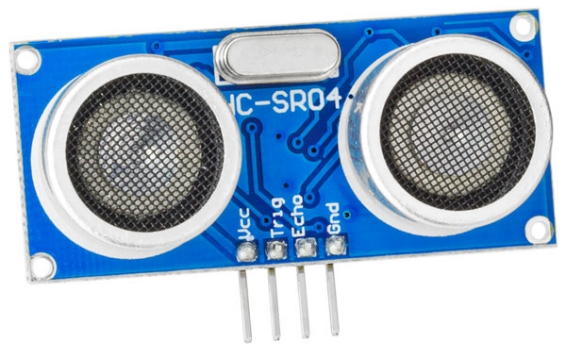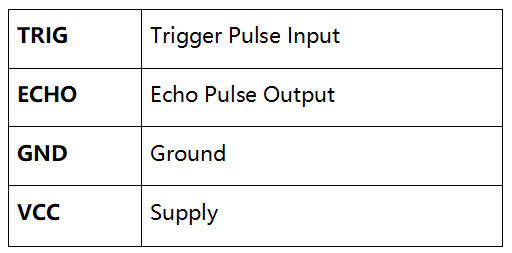The timing diagram is shown below. You only need to supply a short 10us pulse for the trigger input to start the ranging, and then the module will send out an 8 cycle burst of ultrasound at 40 kHz and raise its echo. You can calculate the range through the time interval between sending trigger signal and receiving echo signal.

Formula: us / 58 = centimeters or us / 148 =inch; or: the range = high level time * velocity (340M/S) / 2; you are suggested to use measurement cycle over 60ms in order to prevent signal collisions of trigger signal and the echo signal.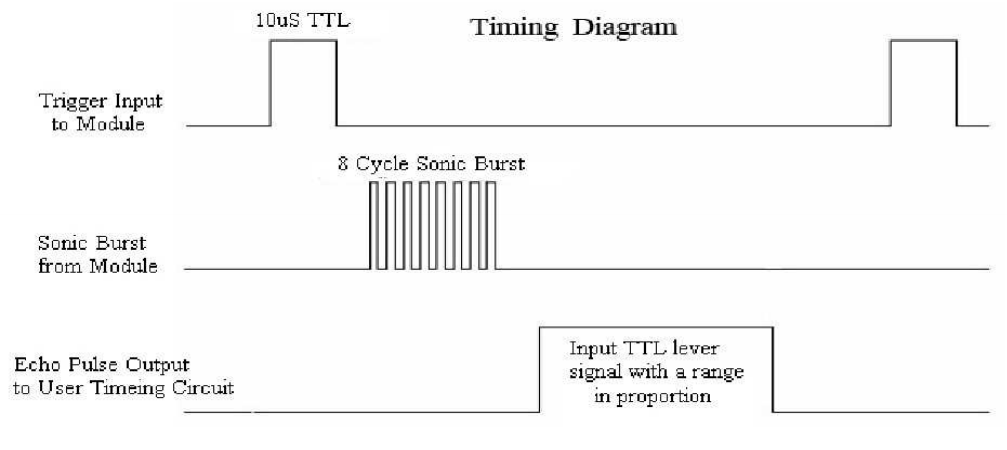## Schematic Diagram¶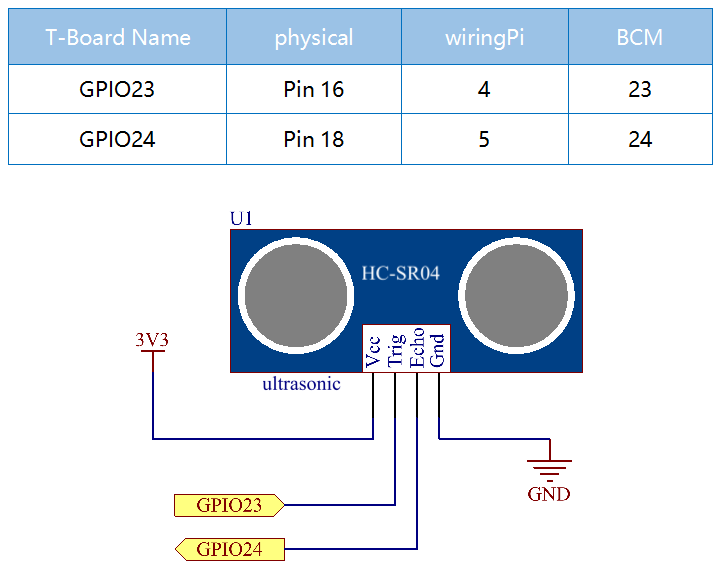## Experimental Procedures¶

Step 1: Build the circuit.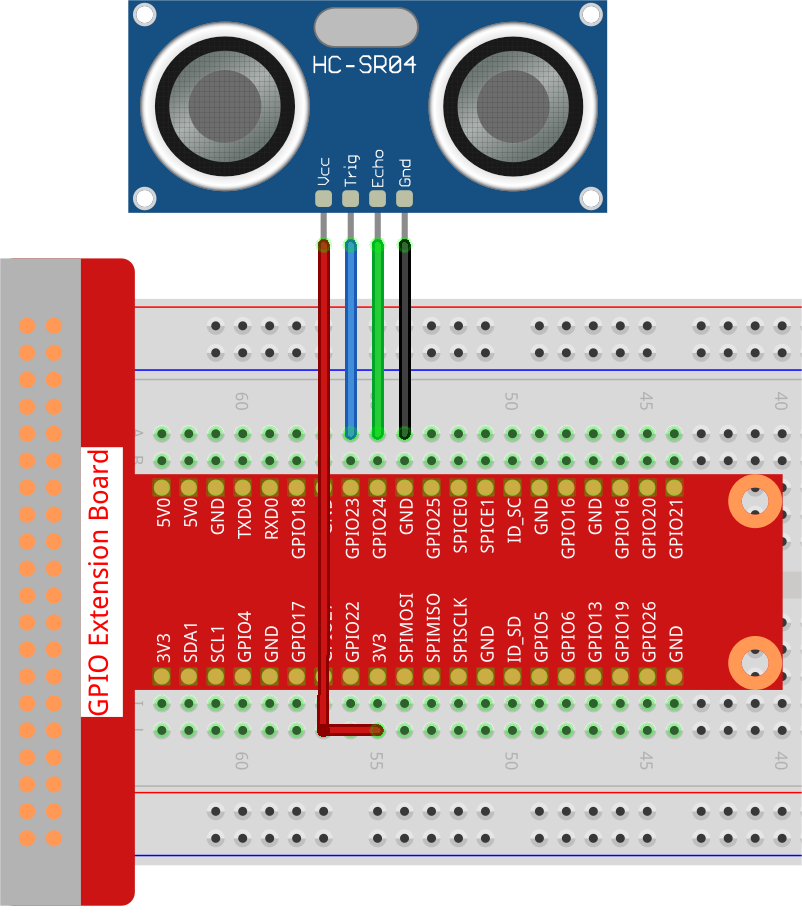### For C Language Users¶

Step 2: Go to the folder of the code.

```cd /home/pi/davinci-kit-for-raspberry-pi/c/2.2.5/
```

Step 3: Compile the code.

```gcc 2.2.5_Ultrasonic.c -lwiringPi
```

Step 4: Run the executable file.

```sudo ./a.out
```

With the code run, the ultrasonic sensor module detects the distance between the obstacle ahead and the module itself, then the distance value will be printed on the screen.

Note

If it does not work after running, or there is an error prompt: “wiringPi.h: No such file or directory”, please refer to C code is not working?.

Code

```#include <wiringPi.h>
#include <stdio.h>
#include <sys/time.h>

#define Trig    4
#define Echo    5

void ultraInit(void)
{
pinMode(Echo, INPUT);
pinMode(Trig, OUTPUT);
}

float disMeasure(void)
{
struct timeval tv1;
struct timeval tv2;
long time1, time2;
float dis;

digitalWrite(Trig, LOW);
delayMicroseconds(2);

digitalWrite(Trig, HIGH);
delayMicroseconds(10);
digitalWrite(Trig, LOW);

gettimeofday(&tv1, NULL);

gettimeofday(&tv2, NULL);

time1 = tv1.tv_sec * 1000000 + tv1.tv_usec;
time2  = tv2.tv_sec * 1000000 + tv2.tv_usec;

dis = (float)(time2 - time1) / 1000000 * 34000 / 2;

return dis;
}

int main(void)
{
float dis;
if(wiringPiSetup() == -1){ //when initialize wiring failed,print message to screen
printf("setup wiringPi failed !");
return 1;
}

ultraInit();

while(1){
dis = disMeasure();
printf("%0.2f cm\n\n",dis);
delay(300);
}

return 0;
}
```

Code Explanation

```void ultraInit(void)
{
pinMode(Echo, INPUT);
pinMode(Trig, OUTPUT);
}
```

Initialize the ultrasonic pin; meanwhile, set Echo to input, Trig to output.

```float disMeasure(void){};
```

This function is used to realize the function of ultrasonic sensor by calculating the return detection distance.

```struct timeval tv1;
struct timeval tv2;
```

Struct timeval is a structure used to store the current time. The complete structure is as follows:

```struct timeval
{
__time_t tv_sec;        /* Seconds. */
__suseconds_t tv_usec;  /* Microseconds. */
};
```

Here, tv_sec represents the seconds that Epoch spent when creating struct timeval. Tv_usec stands for microseconds or a fraction of seconds.

```digitalWrite(Trig, HIGH);
delayMicroseconds(10);
digitalWrite(Trig, LOW);
```

A 10us ultrasonic pulse is being sent out.

```while(!(digitalRead(Echo) == 1));
gettimeofday(&tv1, NULL);
```

This empty loop is used to ensure that when the trigger signal is sent, there is no interfering echo signal and then get the current time.

```while(!(digitalRead(Echo) == 0));
gettimeofday(&tv2, NULL);
```

This empty loop is used to ensure that the next step is not performed until the echo signal is received and then get the current time.

```time1 = tv1.tv_sec * 1000000 + tv1.tv_usec;
time2  = tv2.tv_sec * 1000000 + tv2.tv_usec;
```

Convert the time stored by struct timeval into a full microsecond time.

```dis = (float)(time2 - time1) / 1000000 * 34000 / 2;
```

The distance is calculated by the time interval and the speed of sound propagation. The speed of sound in the air: 34000cm/s.

### For Python Language Users¶

Step 2: Go to the folder of the code.

```cd /home/pi/davinci-kit-for-raspberry-pi/python/
```

Step 3: Run the executable file.

```sudo python3 2.2.5_Ultrasonic.py
```

With the code run, the ultrasonic sensor module detects the distance between the obstacle ahead and the module itself, then the distance value will be printed on the screen.

Code

Note

You can Modify/Reset/Copy/Run/Stop the code below. But before that, you need to go to source code path like `davinci-kit-for-raspberry-pi/python`.

```import RPi.GPIO as GPIO
import time

TRIG = 16
ECHO = 18

def setup():
GPIO.setmode(GPIO.BOARD)
GPIO.setup(TRIG, GPIO.OUT)
GPIO.setup(ECHO, GPIO.IN)

def distance():
GPIO.output(TRIG, 0)
time.sleep(0.000002)

GPIO.output(TRIG, 1)
time.sleep(0.00001)
GPIO.output(TRIG, 0)

while GPIO.input(ECHO) == 0:
a = 0
time1 = time.time()
while GPIO.input(ECHO) == 1:
a = 1
time2 = time.time()

during = time2 - time1
return during * 340 / 2 * 100

def loop():
while True:
dis = distance()
print ('Distance: %.2f' % dis)
time.sleep(0.3)

def destroy():
GPIO.cleanup()

if __name__ == "__main__":
setup()
try:
loop()
except KeyboardInterrupt:
destroy()
```

Code Explanation

```def distance():
```

This function is used to realize the function of ultrasonic sensor by calculating the return detection distance.

```GPIO.output(TRIG, 1)
time.sleep(0.00001)
GPIO.output(TRIG, 0)
```

This is sending out a 10us ultrasonic pulse.

```while GPIO.input(ECHO) == 0:
a = 0
time1 = time.time()
```

This empty loop is used to ensure that when the trigger signal is sent, there is no interfering echo signal and then get the current time.

```while GPIO.input(ECHO) == 1:
a = 1
time2 = time.time()
```

This empty loop is used to ensure that the next step is not performed until the echo signal is received and then get the current time.

```during = time2 - time1
```

Execute the interval calculation.

```return during * 340 / 2 * 100
```

The distance is calculated in the light of time interval and the speed of sound propagation. The speed of sound in the air: 340m/s.

## Phenomenon Picture¶